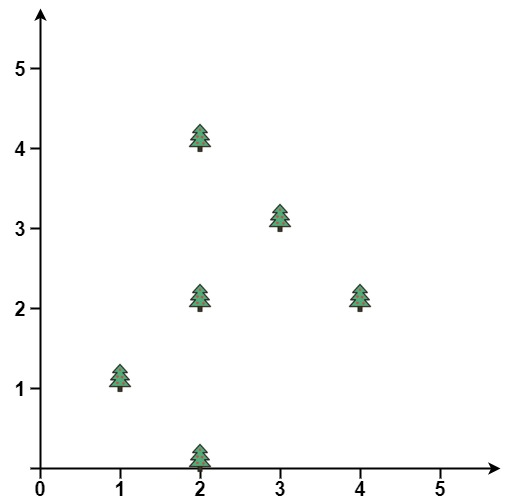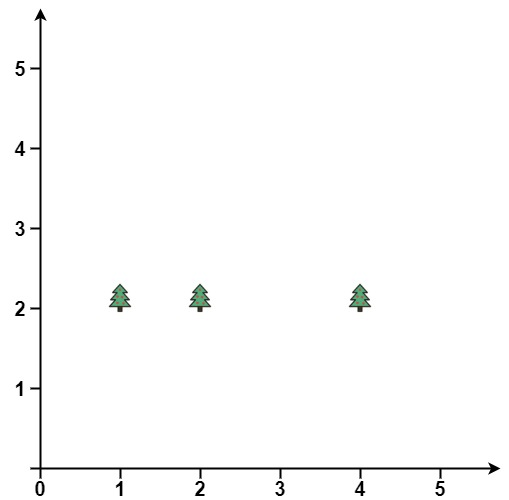# GeetCode Hub

You are given an array `trees` where `trees[i] = [xi, yi]` represents the location of a tree in the garden.

You are asked to fence the entire garden using the minimum length of rope as it is expensive. The garden is well fenced only if all the trees are enclosed.

Return the coordinates of trees that are exactly located on the fence perimeter.

Example 1:```Input: points = [[1,1],[2,2],[2,0],[2,4],[3,3],[4,2]]
Output: [[1,1],[2,0],[3,3],[2,4],[4,2]]
```

Example 2:```Input: points = [[1,2],[2,2],[4,2]]
Output: [[4,2],[2,2],[1,2]]
```

Constraints:

• `1 <= points.length <= 3000`
• `points[i].length == 2`
• `0 <= xi, yi <= 100`
• All the given points are unique.

class Solution { public int[][] outerTrees(int[][] trees) { } }